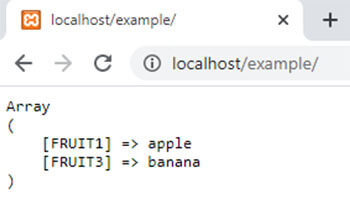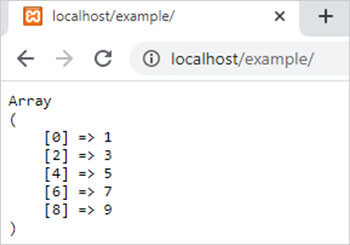array_udiff() function in php || PHP Array Udiff Function# array_udiff() function in php

1579

array_udiff() function of PHP is used to return the difference of two or more PHP arrays by comparing the values of arrays with the help of a user-defined function. It is an inbuilt function of PHP.

Syntax:

array_udiff(\$array1,\$array2,.....,\$arrayN,user_function);

here,

\$array1, \$array2 and \$arrayN are PHP arrays and user_function() is a user define function which contains the conditions which applies on arrays to calculate the difference.

Example 1:

```<?php
function user_function(\$x,\$y)
{
if (\$x===\$y)
{
return 0;
}
return (\$x>\$y)?1:-1;
}
\$arr1=array("FRUIT1"=>"apple","FRUIT2"=>"orange","FRUIT3"=>"banana");
\$arr2=array("FRUIT4"=>"orange","FRUIT5"=>"fig","FRUIT1"=>"pear");

\$result=array_udiff(\$arr1,\$arr2,"user_function");
echo "<pre>";
print_r(\$result);
?>```

Output:Example 2:

```<?php
function user_function(\$x,\$y)
{
if (\$x===\$y)
{
return 0;
}
return (\$x>\$y)?1:-1;
}

\$arr1=array(1,2,3,4,5,6,7,8,9);
\$arr2=array(2,4,6,8);

\$result=array_udiff(\$arr1,\$arr2,"user_function");
echo "<pre>";
print_r(\$result);
?>```

Output:Share:## Big Hosting Days

Upto 75@ OFF on Web Hosting

## Get a .COM for just \$6.98

Secure Domain for a Mini Price